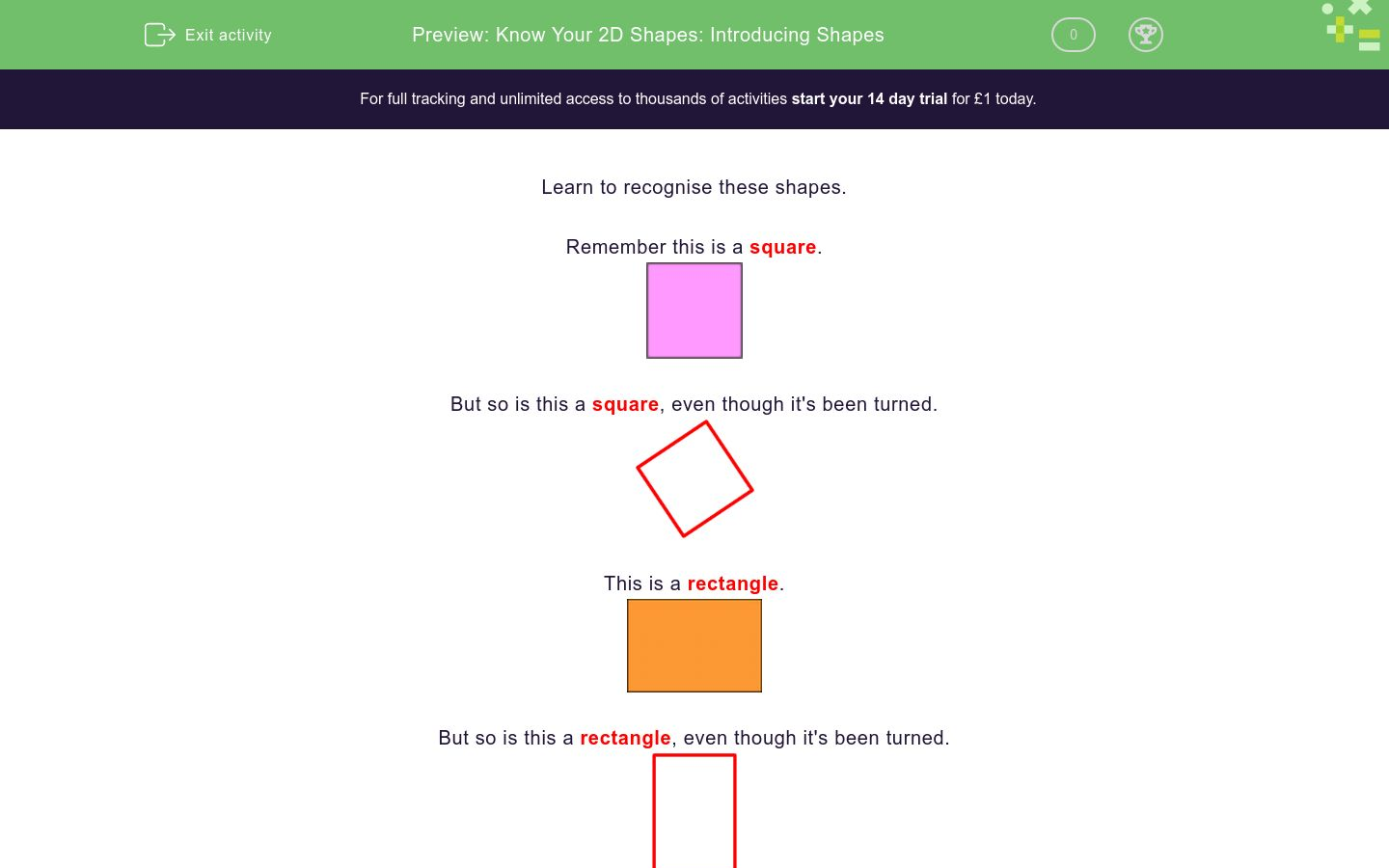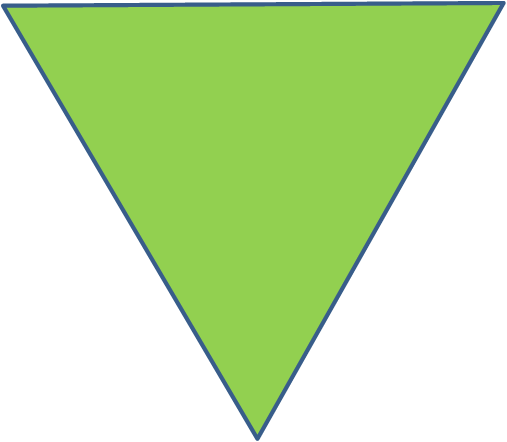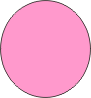# Know Your 2D Shapes: Introducing Shapes

In this worksheet, students recognise 2D shapes and answer questions about them.Key stage:  KS 1

Curriculum topic:   Geometry: Properties of Shapes

Curriculum subtopic:   Recognise 2D and 3D Shapes

Difficulty level:### QUESTION 1 of 10

Learn to recognise these shapes.

Remember this is a square.But so is this a square, even though it's been turned.This is a rectangle.But so is this a rectangle, even though it's been turned.This is a triangle.This is a circle.Example

Is this shape a rectangle, square, circle or triangle?Triangle. (A triangle has three sides.)

Is this a rectangle, square, circle or triangle?Is this a rectangle, square, circle or triangle?Is this a rectangle, square, circle or triangle?Is this a rectangle, square, circle or triangle?Is this a rectangle, square, circle or triangle?Is this a rectangle, square, circle or triangle?Is this a rectangle, square, circle or triangle?Is this a rectangle, square, circle or triangle?Is this a rectangle, square, circle or triangle?Is this a rectangle, square, circle or triangle?• Question 1

Is this a rectangle, square, circle or triangle?Triangle
EDDIE SAYS
It has three sides.
Triangles always have three sides.
• Question 2

Is this a rectangle, square, circle or triangle?Square
EDDIE SAYS
A square has four sides.
The sides are equal.
• Question 3

Is this a rectangle, square, circle or triangle?Square
EDDIE SAYS
A square has four sides.
The sides are equal.
• Question 4

Is this a rectangle, square, circle or triangle?Rectangle
EDDIE SAYS
A rectangle has four sides.
The sides are not all equal.
• Question 5

Is this a rectangle, square, circle or triangle?Circle
EDDIE SAYS
A circle is round.
• Question 6

Is this a rectangle, square, circle or triangle?Circle
EDDIE SAYS
A circle is round.
• Question 7

Is this a rectangle, square, circle or triangle?Rectangle
EDDIE SAYS
A rectangle has four sides.
The sides are not all equal.
• Question 8

Is this a rectangle, square, circle or triangle?Triangle
EDDIE SAYS
A triangle has three sides.
• Question 9

Is this a rectangle, square, circle or triangle?Triangle
EDDIE SAYS
A triangle has three sides.
• Question 10

Is this a rectangle, square, circle or triangle?Circle
EDDIE SAYS
A circle is round.
---- OR ----

Sign up for a £1 trial so you can track and measure your child's progress on this activity.

### What is EdPlace?

We're your National Curriculum aligned online education content provider helping each child succeed in English, maths and science from year 1 to GCSE. With an EdPlace account you’ll be able to track and measure progress, helping each child achieve their best. We build confidence and attainment by personalising each child’s learning at a level that suits them.

Get started